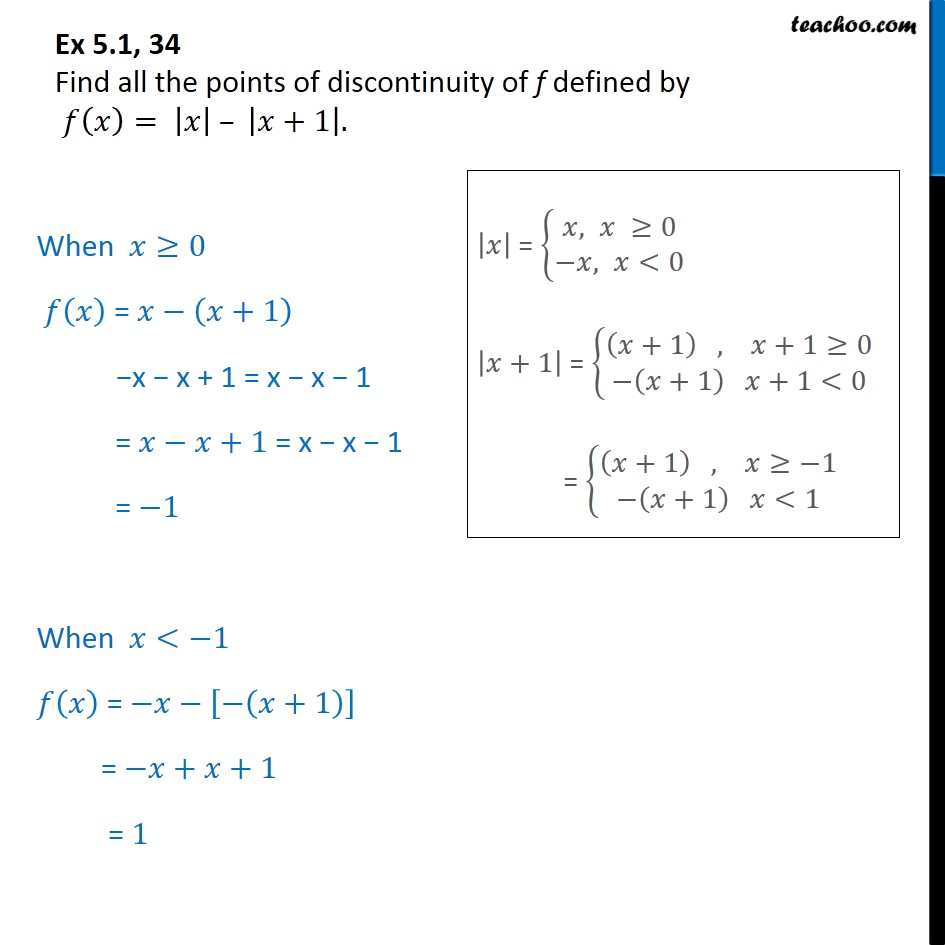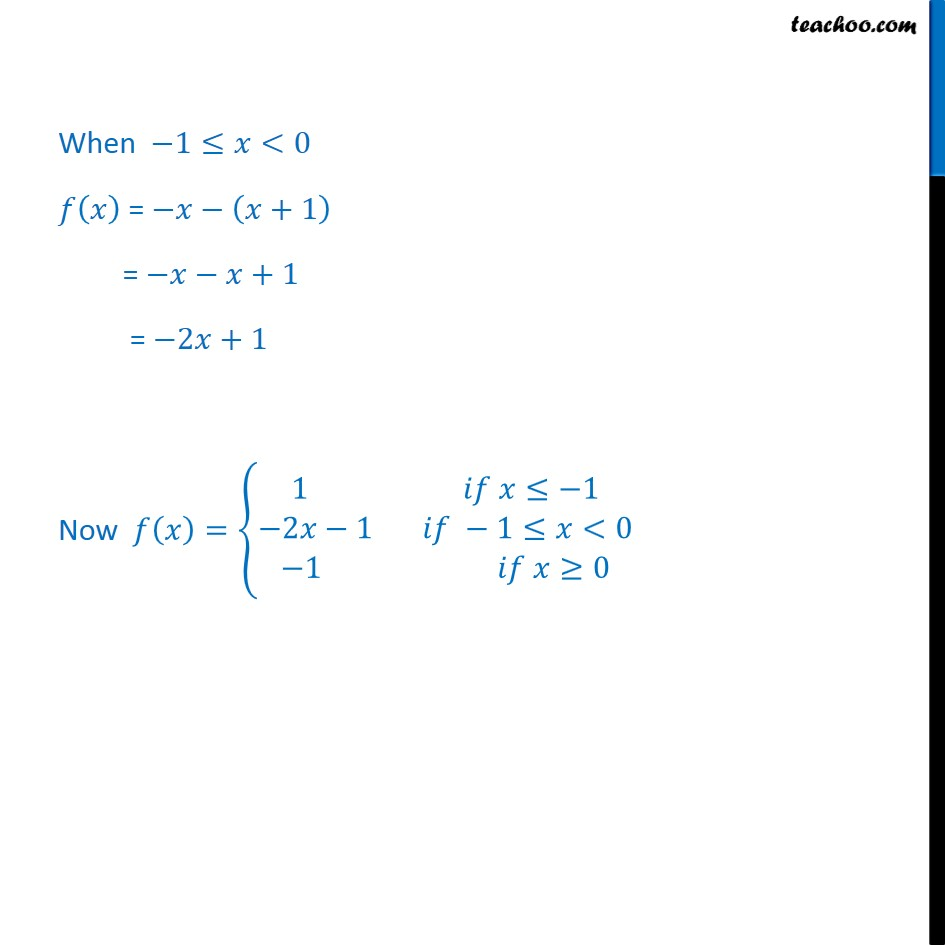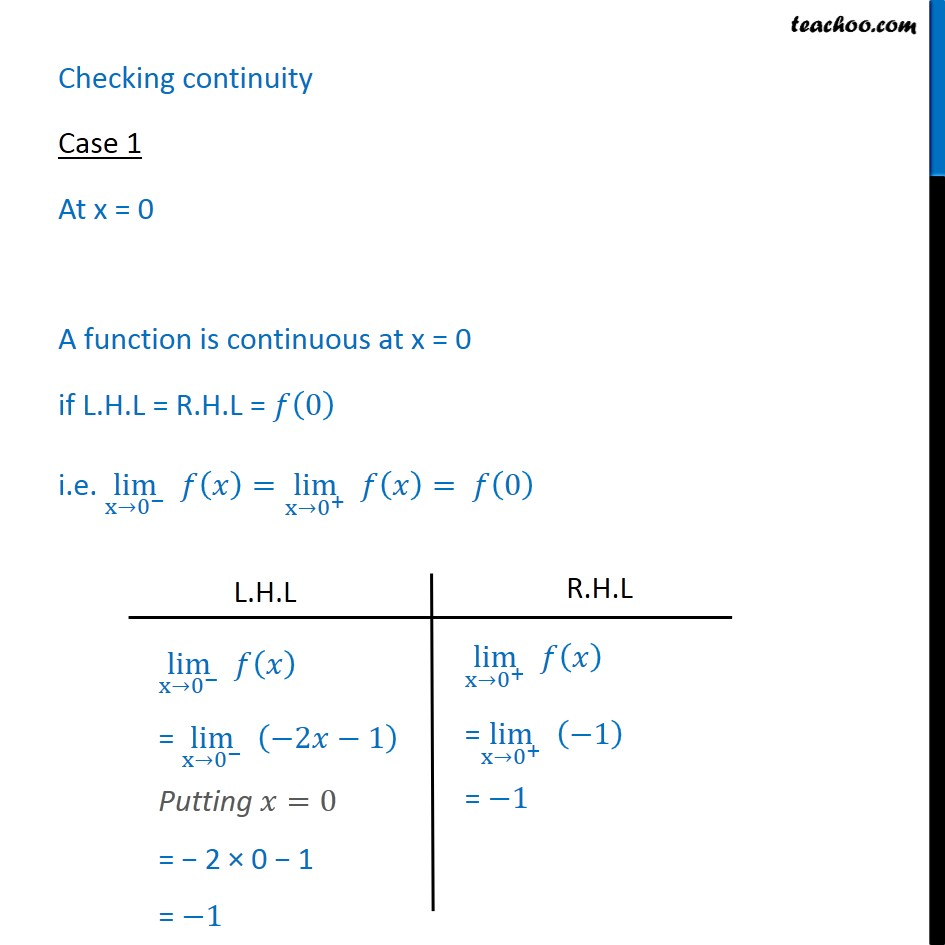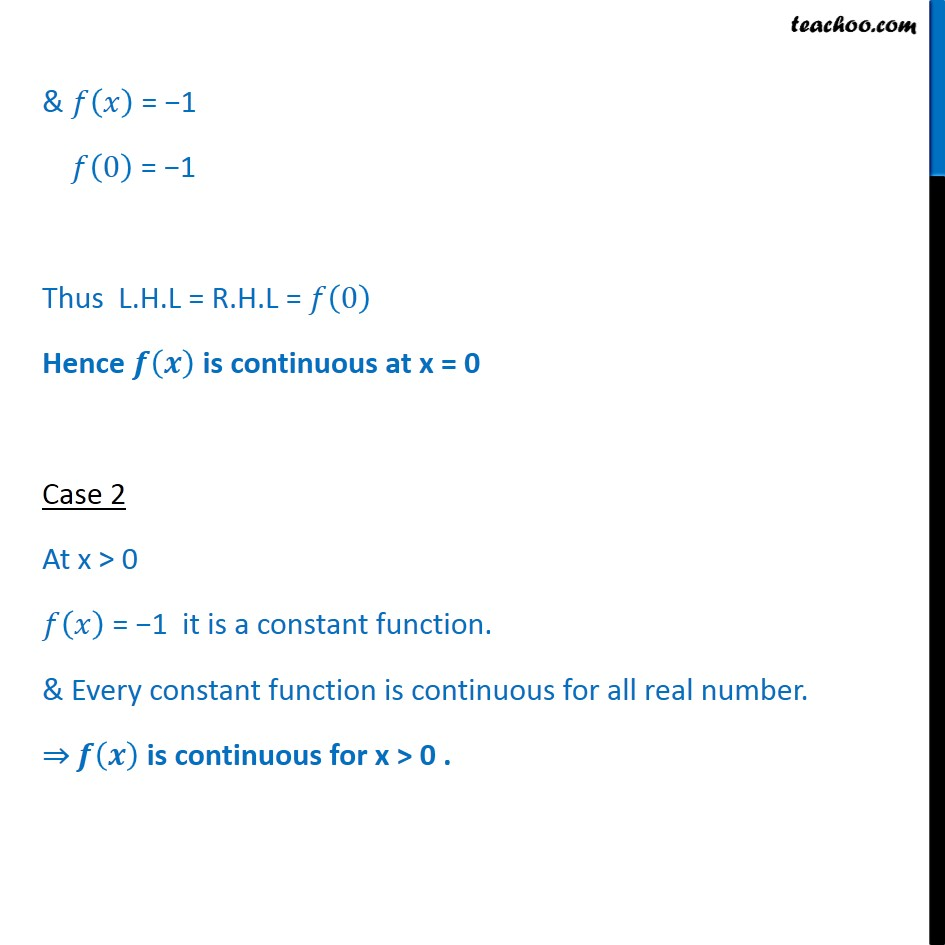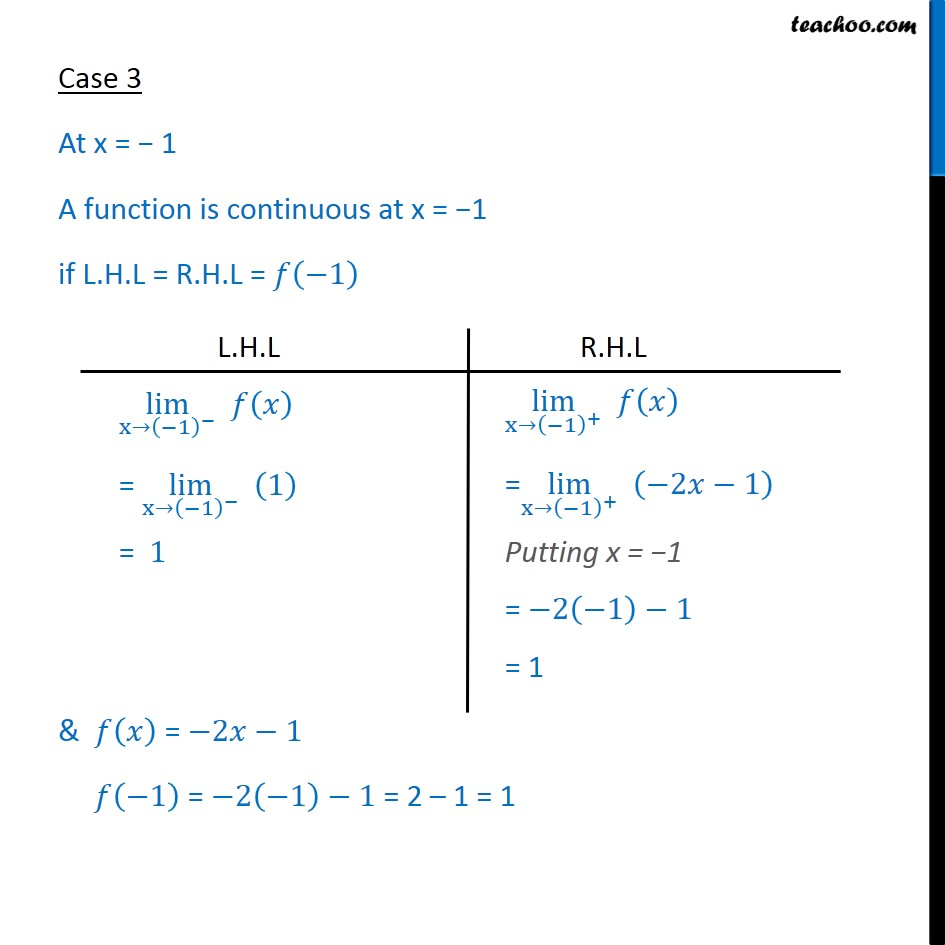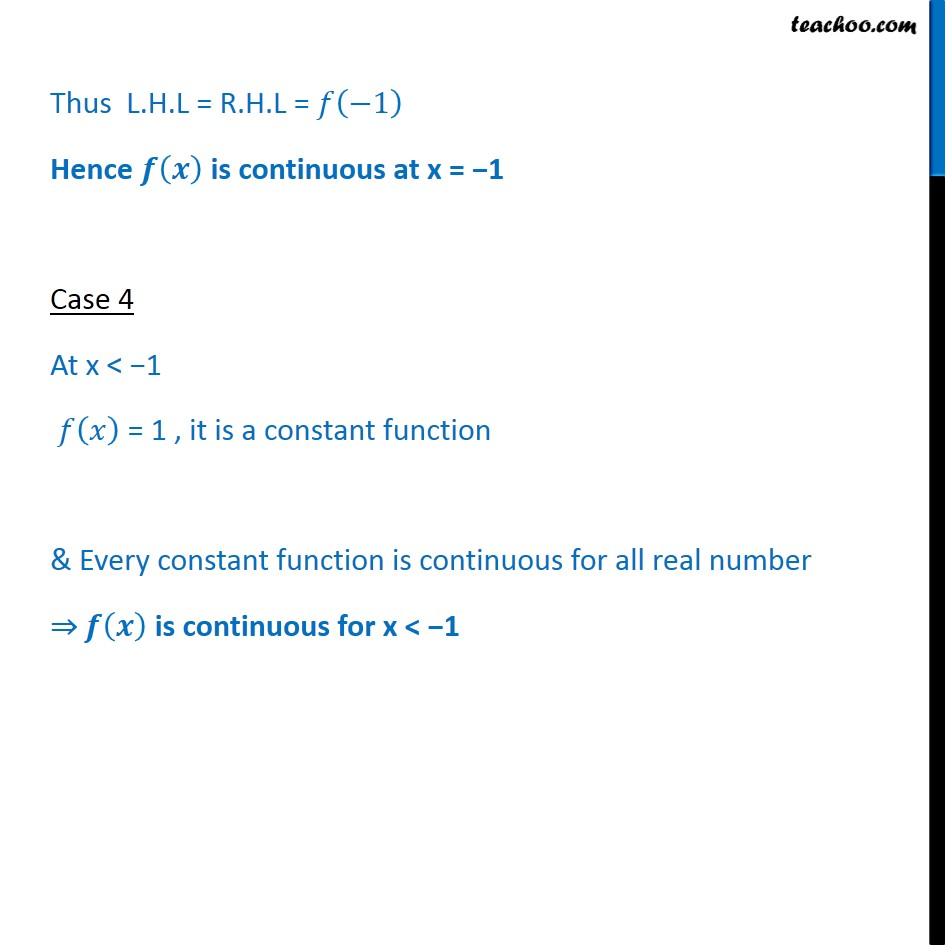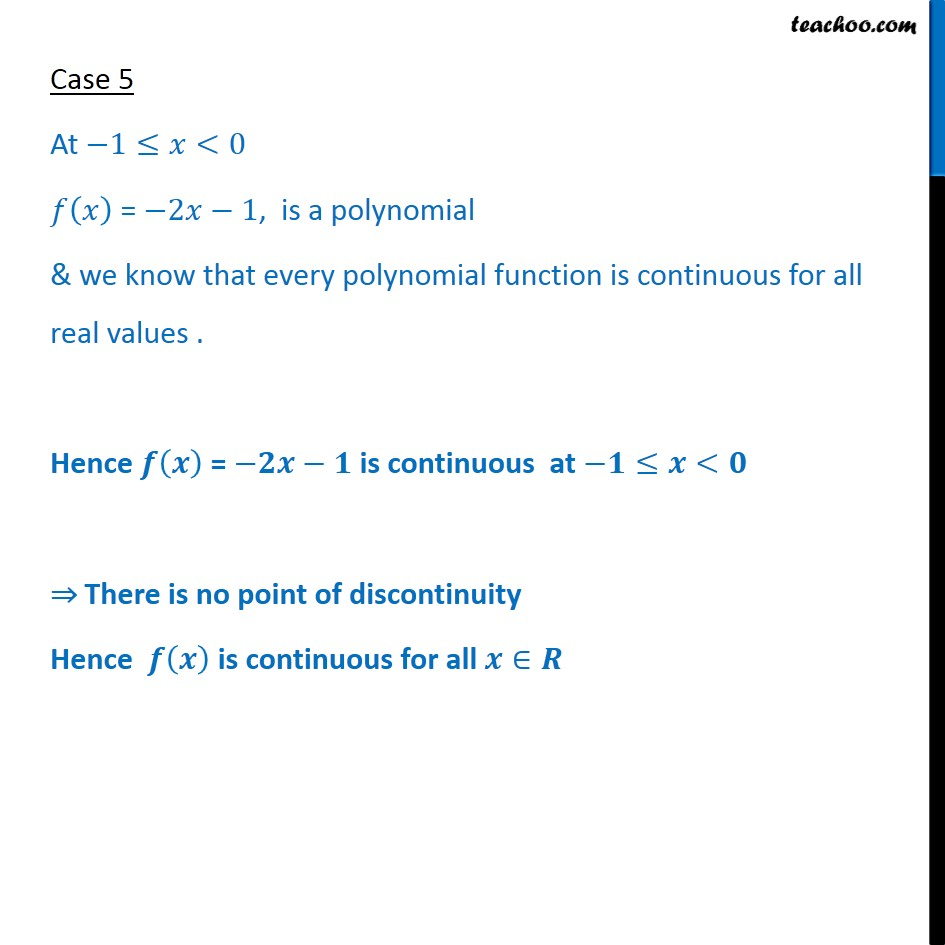1. Class 12
2. Important Question for exams Class 12
3. Chapter 5 Class 12 Continuity and Differentiability

Transcript

Ex 5.1, 34 Find all the points of discontinuity of f defined by 𝑓 𝑥﷯= 𝑥﷯ – 𝑥+1﷯. When 𝑥≥0 𝑓 𝑥﷯ = 𝑥− 𝑥+1﷯ −x − x + 1 = x − x − 1 = 𝑥−𝑥+1 = x − x − 1 = −1 When 𝑥<−1 𝑓 𝑥﷯ = −𝑥− − 𝑥+1﷯﷯ = −𝑥+𝑥+1 = 1 When −1≤𝑥<0 𝑓 𝑥﷯ = −𝑥− 𝑥+1﷯ = −𝑥−𝑥+1 = −2𝑥+1 Now 𝑓 𝑥﷯= 1 𝑖𝑓 𝑥≤−1﷮−2𝑥−1 𝑖𝑓 −1≤𝑥<0﷮−1 𝑖𝑓 𝑥≥0﷯﷯ Checking continuity Case 1 At x = 0 A function is continuous at x = 0 if L.H.L = R.H.L = 𝑓 0﷯ i.e. lim﷮x→ 0﷮−﷯﷯ 𝑓 𝑥﷯= lim﷮x→ 0﷮+﷯﷯ 𝑓 𝑥﷯= 𝑓 0﷯ & 𝑓 𝑥﷯ = −1 𝑓 0﷯ = −1 Thus L.H.L = R.H.L = 𝑓 0﷯ Hence 𝒇 𝒙﷯ is continuous at x = 0 Case 2 At x > 0 𝑓 𝑥﷯ = −1 it is a constant function. & Every constant function is continuous for all real number. ⇒ 𝒇 𝒙﷯ is continuous for x > 0 . Case 3 At x = − 1 A function is continuous at x = −1 if L.H.L = R.H.L = 𝑓 −1﷯ & 𝑓 𝑥﷯ = −2𝑥−1 𝑓 −1﷯ = −2 −1﷯−1 = 2 – 1 = 1 Thus L.H.L = R.H.L = 𝑓 −1﷯ Hence 𝒇 𝒙﷯ is continuous at x = −1 Case 4 At x < −1 𝑓 𝑥﷯ = 1 , it is a constant function & Every constant function is continuous for all real number ⇒ 𝒇 𝒙﷯ is continuous for x < −1 Case 5 At −1≤𝑥<0 𝑓 𝑥﷯ = −2𝑥−1, is a polynomial & we know that every polynomial function is continuous for all real values . Hence 𝒇 𝒙﷯ = −𝟐𝒙−𝟏 is continuous at −𝟏≤𝒙<𝟎 ⇒ There is no point of discontinuity Hence 𝒇 𝒙﷯ is continuous for all 𝒙∈𝑹

Chapter 5 Class 12 Continuity and Differentiability

Class 12
Important Question for exams Class 12